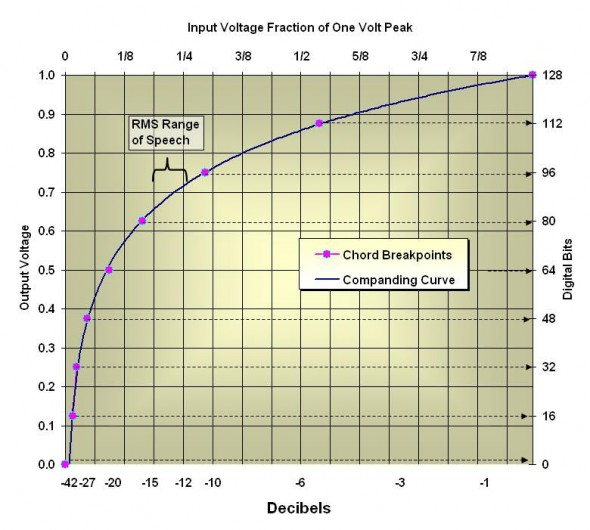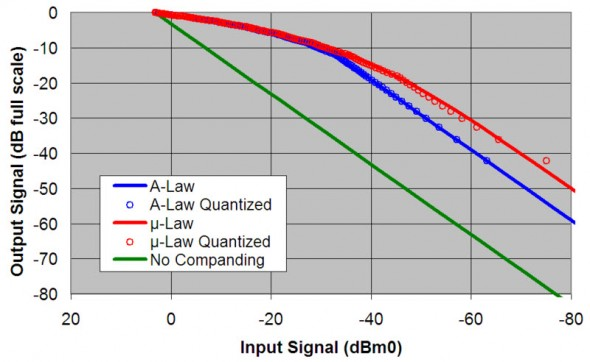# The Squeeze – How to turn 8 bits into 15 bits and get away with it!

Campbell’s Corner – By Dick Campbell

## Dick Campbell asked the question,  How to turn 8 bits into 15 bits and get away with it? He will tell you how in the article.

Anyone listening to 8-bit linear PCM encoded audio can readily hear something distasteful. It’s a bit ragged at best. Linear pulse code modulation (PCM) simply divides the entire dynamic range into 28 -1 or 255 equal quantization intervals. One bit of the eight must be devoted to the sign bit to signify a positive or negative analog swing. That leaves only seven bits or 127 quantization intervals for the instantaneous waveform, either positive or negative swing. The ½ bit left over vanishes into the swamp!

For example, if the instantaneous signal is a one volt peak , then the smallest interval nearest zero, called the least significant bit (LSB), is 1/127 volts or 7.9 mV. So the signal has to reach this level, ~ -42dB peak, before the ADC (A-to-D converter) switches to the first count above zero. However, we wish to encode real audio signals, not sine waves, thus we are faced with peak-to-RMS ratios of ~15dB for speech. If peak clipping is not allowed, then the true ‘RMS-available’ dynamic range is 42-15 = 27dB. Yuk! And that’s ideal – even the telephone engineers would not put up with that!

The solution to making it sound better is to use some form of compression by defining a non-linear coding scheme. Put more bits in the lowest part of the dynamic range, and fewer in the highest part. If that sounds like analog compression/expansion you would be correct. Squeeze it, transmit it, unsqueeze it – making 8 bits “sound like” 15 bits to the listener.

We can thank the telecom engineers for standardizing this . When you hear a “toll-quality” long-distance call you are most likely listening to a special encoding that is called μ-law (in the USA and Japan) or A-law (the rest of the world), each with slightly different encoding equation. International calls always fall back to A-law if that is used at one end. Most of the development found in a patent search dates for the mid-fifties, however I found one reference to a 1937 patent on signaling (not available on-line).Figure 1. Companding curve for μ-law encoder showing piece-wise linear break points for one-half of the instantaneous amplitude range .

Figure 1 illustrates a logarithmic encoder that uses eight straight-line approximations to the curve. Note that each straight section, called a “chord” uses 16 bits of the 128 bit range (actually 127 because the “0” bit is reserved for sign). Such a convenient combination! Eight chords, each one with 16 bits of data. That’s three binary bits to define which chord we are on, and four binary bits to define where we are on the chord. Seven bits plus sign!

Send those bits along then reverse the procedure at the other end and we have a high-quality telephone signal where, effectively, one-half of the digital dynamic range is used to describe the lower one-tenth of the input signal range. The sample rate is 8000s/s so a bandwidth of about 64 kb/s is required. Due to its relatively low RMS, the speech signal transforms into bytes with lots of zeros making clock reconstruction at the receiving end problematic and subject to interference. Inverting the bytes to push mostly all ones solves that problem.

One application of this I have used is speech message storage. Storing 8-bit words of μ-law encoded messages widens microprocessor selection possibilities. Even the simplest 8-bit device can restore high-quality speech in real-time via a SPI port. In fact, μ-law is so ubiquitous that the code is readily available for most processors.

Piece-wise linear transformation is an old and powerful technique in discreet approximation to an analog curve and found in numerous signal processing routines. dcFigure 2. From: http://en.wikipedia.org/wiki/ Mu-law_algorithm this illustrates  how a 70dB signal dynamic range can be squeezed into 50dB with Alaw and 40dB with μ-law. The small open circles show deviations due to piece-wise approximation error.

 M-law algorithm. (2008, March 23). In Wikipedia, The Free Encyclopedia. Retrieved 15:03, March 31, 2008, from http://en.wikipedia.org/w/index.php?title=%CE%9Claw_ algorithm&oldid=200278749

 This is a nice introduction to DSP and applications.

http://www.ece.rochester.edu/courses/ECE446/lectures/Lect ure1.pdf

 Note that Fig. 2 is from Wikipedia which is a collaborative open-edit source, not from a peer-reviewed paper.

 For a deeper look into the coding algorithms and other technical data. Browse the International Telecommunications Union (ITU) in-force recommendation G.711x : http://www.itu.int/rec/T-REC-G.191-200508-I/en

Tags: